#0
0
01. 云栖社区>
2. 博客>
3. 正文

## 前端优化系列 - JS混淆引入性能天坑

atuanxy 2018-07-30 10:48:38 浏览2810

# 混淆引入性能问题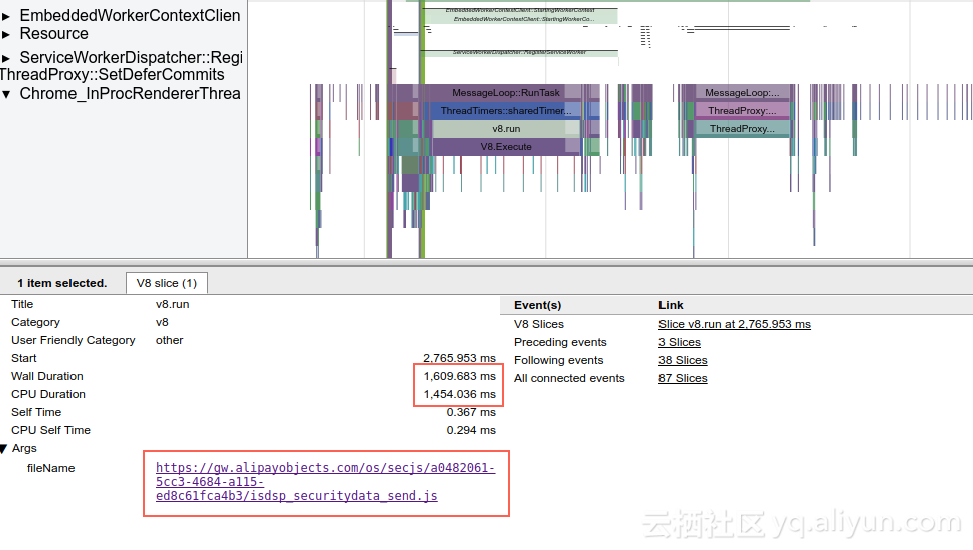# 分析性能问题

（1）确认性能问题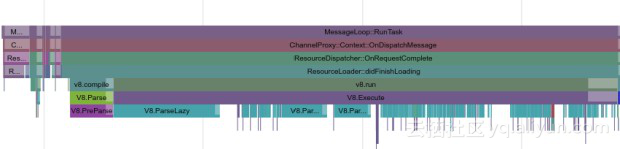V8.Execute 代表v8内部的JS执行时间，与v8.run代表的意义一样，耗时也相近。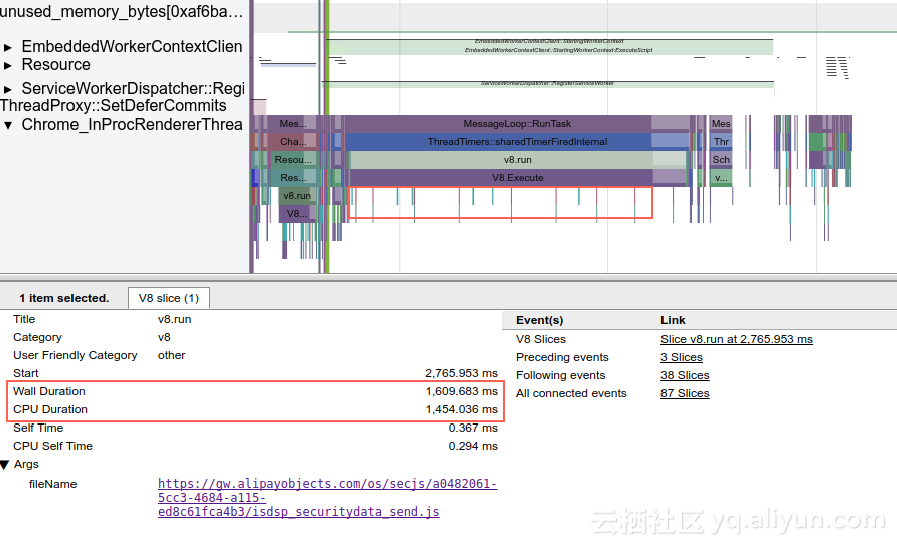（2）分析问题原因

 `` `` `` `` ``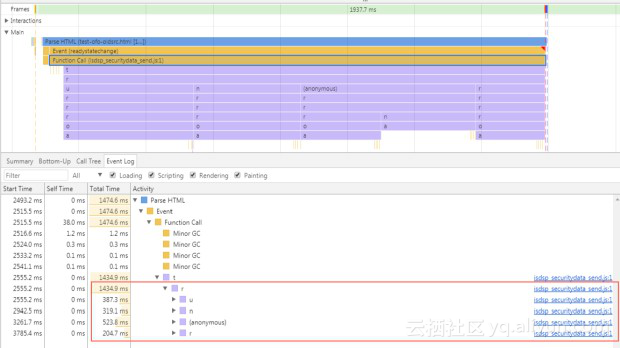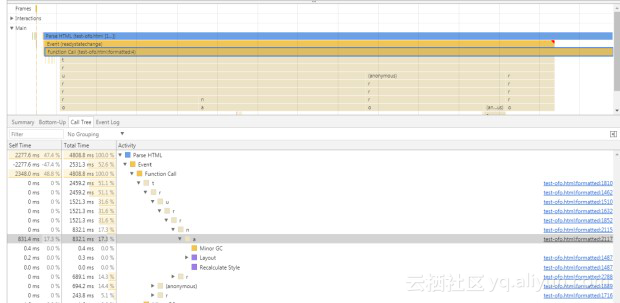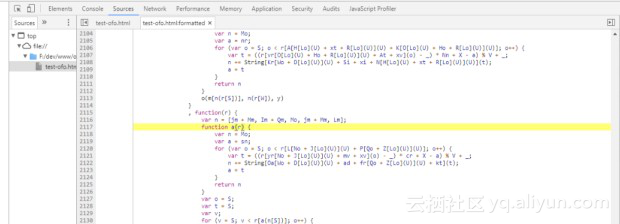`function` `a(r) {` `  ``var` `n = Mo;` `  ``var` `a = sn;` `  ``for` `(``var` `o = S; o < r[L[No + J[Lo](U)](U) + P[Qo + Z[Lo](U)](U)]; o++) {` `    ``var` `t = ((r[yr[No + J[Lo](U)](U) + mv + xv](o) - _) * cr + X - a) % V + _;` `    ``n += String[Oa[Wo + D[Lo](U)](U) + ad + fr[Qo + Z[Lo](U)](U) + kt](t);` `    ``a = t` `  ``}` `  ``return` `n` `}`

JS字符串拼接的性能为什么会很差呢？

``````var combined = "";
for (var i = 0; i < 1000000; i++) {
combined = combined + "hello ";
}``````

 `` `` `` `` ``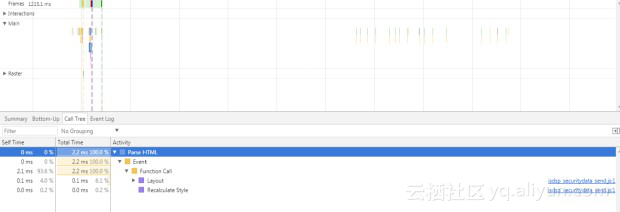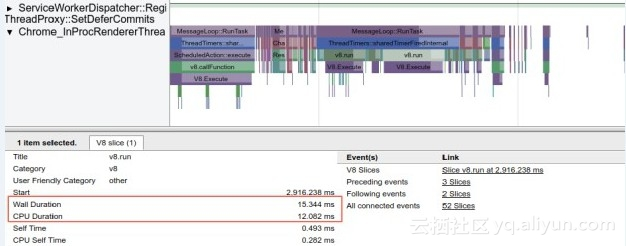# 结束语

（1）将一些通用的前端分析方法整理成文档，供前端参考。

（2）将一些人工分析总结的经验，固化到自动化的工具，比如，WDPS Lighthouse。

（3）提供一些更有效的分析工具。比如，在Trace中更清晰的展现JS引擎的运行逻辑。

（4）与前端更多交流合作，建立互信，深入合作研究疑难问题和普遍问题。

# 参考文档

Why is + so bad for concatenation?

Optimization killers

atuanxy
+ 关注